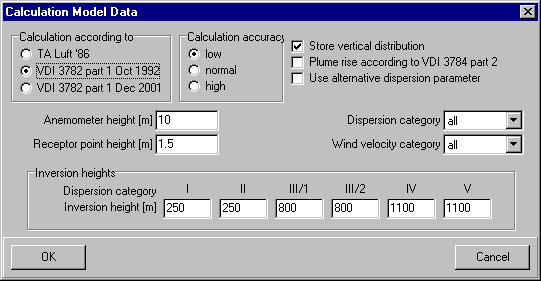# P&K 3782

## Calculation model data### Calculation according to

Dispersion calculations according to TA Luft 86 and VDI 3782 part 1 can be performed with this program. The program functions are identical for both models. (Information about ground roughness, sedimentation, and mechanical lift of the waste gas plume are meaningless in the TA Luft model). (more)

When calculating according VDI 3782 part 1 / 2001, the 9 TA Luft wind velocity categories are taken into account, as well as a new parameter set for 180 instead of 150 Meters in the dispersion parameter calculation (F, f, G, g).

### Calculation accuracy

These settings influence the calculation accuracy and therefore the calculation time and the needed disk space. (more)

### Store vertical distribution

Characteristics for receptor points of different heights or graphical cross-sections through the waste gas plume are possible with stored vertical distribution only. (more)

### Rise according to VDI 3784 part 2 {With the option cooling tower only}

The calculation of the plume rise will be performed with the Model VDI 3784 part 2. The accompanying form will be opened. The program VDISP.EXE distributed by VDI has to exist in the program directory. (more)

### Use Alternative Dispersion Parameter

Dispersion parameter deviating from the guideline can be used. The accompanying form can be opened with this button. Parameters will appear on the print-out of characteristics calculations. (more)

### Anemometer height [m]

The anemometer height is to be entered between 3 m and 50 m. The knowledge of the anemometer height (in rare cases deviating from 10 m) is required for the correlation between the vertical wind profile and a wind velocity class. For very low sources VDI 3782 defines a constant wind velocity from a height of 10m. As within the context of TA Luft such an agreement does not exist, this program assumes a constant wind below 3 m here.

### Receptor point height [m]

Set the receptor point height between 0 m and 100 m. For a certain receptor point height, concentrations are always entered. If a concentration array without vertical distribution is calculated, the receptor point height is the only available height during the characteristic calculation (usually the receptor point height is 1.5 m). (more)

### Calculation for dispersion category

The concentration area calculation can be limited to a dispersion category. Therefore the calculation of the characteristic statistic is also limited to this category. (more)

### Calculation of wind velocity (1-9 and for VDI 3782 part 1 1992, 1-15)

The concentration area calculation can be limited to a wind velocity category. Therefore the calculation of the characteristic statistic is also limited to this category. (more)

### Inversion heights

Inversion height values can be entered between 250 and 1500. Dispersion calculations according to VDI 3782 part 1 consider the existence of an inversion barrier layer impenetrable for the waste gas plume. (more)

Language: